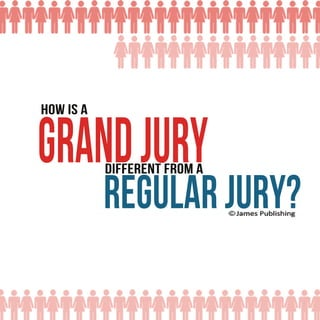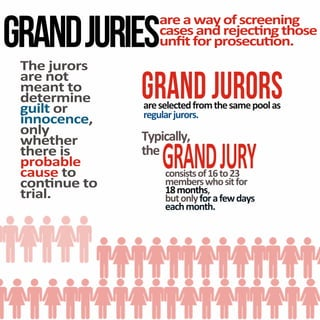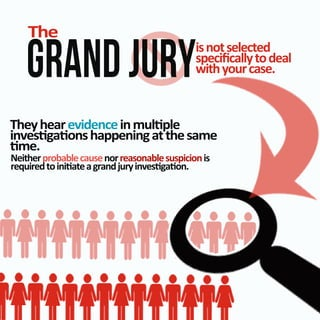Ce diaporama a bien été signalé.
Le téléchargement de votre SlideShare est en cours. ×

# How is a grand jury different from a regular jury?

Publicité
Publicité
Publicité
Publicité
Publicité
Publicité
Publicité
Publicité
Publicité
Publicité
PublicitéPublicité
Publicité
Publicité
Chargement dans…3
×

## Consultez-les par la suite

1 sur 17 Publicité

# How is a grand jury different from a regular jury?

There are differences between a grand jury and a regular jury. Grand jurors are selected from the same pool of regular jurors, they hear evidence in multiple investigations happening at the same time. They are not impartial bodies. Your attorney will not play any role in the challenging or selection of the grand jurors, as opposed to the regular juror selection process. The grand jury proceedings are secret, and you will not be present when other witnesses testify or evidence is presented to the grand jury.

There are differences between a grand jury and a regular jury. Grand jurors are selected from the same pool of regular jurors, they hear evidence in multiple investigations happening at the same time. They are not impartial bodies. Your attorney will not play any role in the challenging or selection of the grand jurors, as opposed to the regular juror selection process. The grand jury proceedings are secret, and you will not be present when other witnesses testify or evidence is presented to the grand jury.

Publicité
Publicité

### How is a grand jury different from a regular jury?

1. 1. Hws oi a gaduy rnj r df e f m ie n r a fr t o rgl j y eu ru ? a r
2. 2. G NJI R DRS A UE a a a osen g r w yf r i e cen c eade cn t s a sn re gh e s j o uﬁf p s uo. nt rr e o oc n Teuos hj r r a nt r o e m ato en t dt mn e ri e e gio ut r l i oec, n cne n ol n y we e ht r h t ri h es e poal rbb e cueo as t cn neo o ut ta rl i. Gaduos rnj r r a slt f mt s e ols r e c dr h a poa e ee o e m r ur rs e l jo . gau r Tp ay yil, cl t h e GR J Y AND UR cnisf6o 3 osto1 t2 s m m e w oio e br h sf s tr 1m n s 8 ot , h btn f ae dy uol o f as yr w ec m n . ah ot h
3. 3. Te h gaduy rnj r i oslt s te c d n ee sei ayo el pc cltda ﬁ l wtyucs. i ora h e Te haei nen u p hy erv ecim l l d e i e g osapn g t ea e n s a nhpei at s v n h m m. e N iep bb cue or snb ssi ns ehrr al asnr a al up i i t o e eo e c o r ue ti a a r du i e g o. e idon t g n jy vs a n qr i e a rn
4. 4. Gadui rnj e rs aeoi atl rnt p i m ra bds oi. e Te haol h ei n t hy ern t v ec h y e d e e p s u r os tp s t r e t c o s re , o co h e o e n m c oi er y t f mo u f ha a i h o h t s n e r f ie i su m r d y w n re s t v w m az b l i e a ef c etgn . n r m naet oe s Yu or atre tony d e n t rsn eie c i y u o s o pee t v nen o r d d fnea d o d nt ae o ee s,n y u o ’h v t tsf,h u h o cn h oe o e yto g y u a co s t d s. oo
5. 5. Yr o u atre’rl tony oe s i hg nj y n er du t a r p c dg r e is o en U le nk i r dfajny sopa ay e l u rn y g rr i u o, yu e ne o e wl t l n o r es a r l I n r en hlni o sl n t o icaeg g re c gh l l n e e g n jo . r du r a rs a e a oao w mns t ,oraete lee t ayt elcmpn ntcwt o a scy sr hil nd a y rl s i drgr du un g n jy i a r t m n. e oy s i esa e nt r t t 1 0
6. 6. Ee w e vn hn alwd loe mssaepoit otttsrh i b cuslrm b ci t, onefo ojtgo en a u g i,rusoi wt s s r r i wt oqe n g ies , gn h n n eo iay a prc an ecptave n n w y a i g x t di p e o s t i ln . h ri t ec s e I n teetts hssae, ihri n b co f e s oj n t ea e tb m d oi o e ae r a f qe o m sb us n ute cre,or o e li dyua r y aﬁ n m sw i et ut h pr e s h i t coso o ad n r ntyu n su yu i aeh o wl k t l m e oj o or us b c n r qet e e t crc o. h li a n e aﬁ i esa e nt r t t 4 0
7. 7. Io fu yr atre t ny o i oa oetb s tl wdoe n l p s t h y t ty r e we o e i, en n u sf y c sll va eau tnn o a tle ef r q soad u n i a t ei c s t i c n le rasen. o u w ho s b o n r g n l t u e fe w i Bto m y e uyu a b rs ie etc d rt ihwo e yu no no m y osl i a cnu wt t h yu l yr o or w e. u a Y wl ato ae iw n t sv l te potn yo h opr i t ut cnu fr osl o: t
8. 8. Qetn usis o yu n yu o ad or a ony ae re hv dc e iavne ei dn dac d a sni e r es v. e U epc d nxet e qe os us n. Bfrte rcei syu a ony e eh poed g,or re o n wl rpryu y si vr os f ipea o b ak g aa n o l e n i qe osh poeuo m y s. us n te rsctr a ak Whn o’ pea do m sote e yu e rpr fr ot fh r e qe osyu a sv yu opr n y us n,o cn ae or pot i ut t cnu fr noeenhsl o o osl o ufrse,o e r t , cnui qe os ofs g us n. n
9. 9. Ternjyrcs hgadu poes r Gaduy rcei s rsc t r j poed ga er . n r n e e Yu int e rsn w e ohr i esse fo o wl o b peet hn tewt s ts y r l n e ei nes rsne t te r duy v ec i eetd o h g n j . d p a r I o tsfate rcei s f u e y th poed g, y n te rsctr ibi yuno h h poeuo wl r g o i te l n t g n j yom r du ro . a r Yu iw lt tern oaom o wl a o h f t f ro l k o ad i rt dnrn oteuos n s o s n if t fh j r t a o r .
10. 10. Yu ig e or o wl i yu lv T T OYdrah E I Nu eot, SM n adfo l yu if e r i lea e n i ui o wla cmn pnl s y e lc i a fr ej y ra e t e et o pr ro fs s tm ns u l a . Yu a rueo nw r qe o o cne stas ea us n f i h as ew u tn t i r i tyu f e nw r ol ed on i n e o, t d cm a ad o wl e em e a n yu ib pr i d l “ laeadtlslwi r n y resnb nut t t i” e o ac o p u t a e po t v n oo o h cuslui te onedr gh n qe oi . us n g n
11. 11. Te h psur r et oco wl uso yun m ner e bn t lreoi n i e n o ia anr sm lgr odps o l q e i i a qe oi , t iote neonetojtr j g tievn. us n gb wt udf scusl b coau eon r e n u h e o e d t e eo rr r I o m ka f u ae n y w i tsfn ( i nes n a uso, hee y gms drad qe n l i u t as ei o p tl ec, nw r cm le ,t) n e y . yua o e cnocr ci n or r yanto et o n r t r ic sb cuos hn e r to ea uw e de , t fn. e yg s i
12. 12. B sryu eu o e u et d n ra dsn t qe o, h us n e ad nw rn t qe o a e, l n as eol h us n s dfl ye k uy eog tb udrodbto o . nuho e ne t ,un m r so e I o hv ay obata f u ae n dutso y qe o’ en g a yu o us n m ai , yo d s ns ntne t d oudra . sn
13. 13. Yu a b a e to e o cn e s do ﬀr k hasynoios eraadpi . nn Ep it et tfornweg ad xlnh x noyuko l e n a e e d t su eoi h or f t e c r. I o hv a iprc f u ae nm eet y f m m raotn vn e o bua eet y , p f e ornw ry ra yuas eb ec ep in t xln gh ai e l i osn or i t no yu ma ko l g: nwe e d
14. 14. “ o’ In d t ree emr m b t eat o ssdbtko I et h xcw r I i uInw w n e d a, aed n bl am bstd e ha ad i d s y oso m . l e l ” “ o’ In d t kcwhn e nlw e h o eat x y sdt uJ td a ibt eo i, o l m tt e eh a r a St dy e ol ar w wu ua d ntaeo o y ohv tw r r aot oe bum ny ay o . nm r” e
15. 15. Dl ei ce n i i osopcle rus n t ntseu toges va a . “ o’ nww aJ w sh k go“ I n ko ht e atni ” rI d t o in dn r e bra acp b o’e m e r c t l tm ” e e ae as e —ir . nw r f u s te Prrp sc osae oeo eu r eu nhv gn t jy o cniealases oIoccl nw r, vt o n oe“ n r ” vr d ’ sdn s nw lh g n o o’t e at r d to le a jy i at g fIo’ u wt si o“ n r h r n d t r a”nw r e las e . cl s
16. 16. psur r et oco Whnh et e t g n jo cn sqe os h r du r a a us n. e a rs k ﬁi e, n hs s Sme rsctr o poeuos wlx syu hehya eadc e t qe os t r i c e o w it gt rn senh us n; h s l u e l e h r e oe wl e ih jo ta ayuihr i r t eu r o s so st e l m t rs k p te. Tee usosa b epcl hsqe ncn e seil ay dne ubcuehy e t e agr seast o na o e k t f moacs os h o fc a n. er u Ee i C U INn nw rghi xrs A T ias en t r ce O i e qe os n cnu wtyu us n, d osl i or a t h a o e w e ncs r r y hn ees y n a.
17. 17. Iimn nc e dt t A r du i im n g n jy d t et a rn c dent en o’ be f n gi osom a yu e eno d ut v u l y oar ei n m ash jo f l f cm ; ol ent u r e i t y e rs e t ri nuh v ectca e o h es og ei neo hr yu e e d g wtar e n p ce tt l i cm ad r edor . h i o i a Tea wl erd h cs i t e l i b e bf e d e njy e ra i r t r o ﬀ e u, ad or o e wl r et n yua r y i e n n l s p ei ne n t m n iyu v ecade oy or d s n df s. e ne e